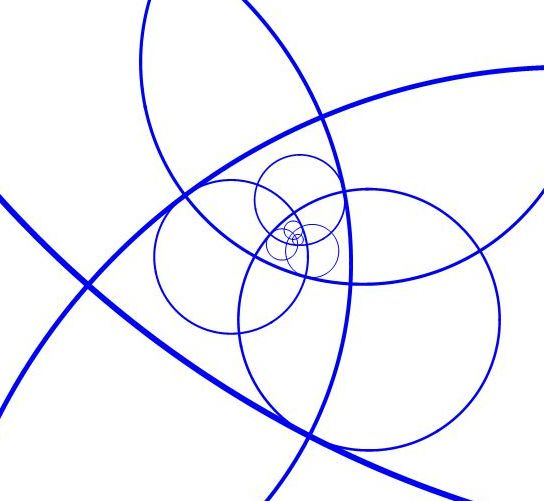# Fractal Orthogonal Circles

Geometry Level 5

Let $R>1$ be the ratio of diameters of successive circles in an infinite self-similar sequence.Let $n$ be any $n$-th circle in the sequence. Then the following statements are true:

• Circle $n$ intersects circles $n-2, n-1, n+1, n+2$ at right angles and no other circles.
• Circle $n$ is tangent only to circles $n-4, n+4$, at points where they intersect circles $n-2, n+2$ respectively

Find $\left\lfloor 10000\times R \right\rfloor$.

Bonus: Find exact answer, which is short and pretty.

Note: A self-similar geometrical sequence is congruent to itself, after scaling, translation, and rotation.

×